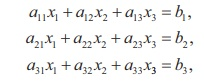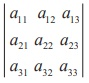Home | | Maths 12th Std | Matrices: CramerŌĆÖs Rule

# Matrices: CramerŌĆÖs Rule

This rule can be applied only when the coefficient matrix is a square matrix and non-singular.

CramerŌĆÖs Rule

This rule can be applied only when the coefficient matrix is a square matrix and non-singular. It is explained by considering the following system of equations:where the coefficient matrixis non-singular. ThenLet us put ╬ö =. Then, we haveNote

Replacing the first column elements a11 , a21 , a31 of ╬ö with b1 , b2 , b3 respectively, we get ╬ö1. Replacing the second column elements a12 , a22 , a32 of ╬ö with b1 , b2 , b3 respectively, we get ╬ö2 . Replacing the third column elements a13 , a23 , a33 of ╬ö with b1 , b2 , b3 respectively, we get ╬ö3.

If ╬ö = 0, CramerŌĆÖs rule cannot be applied.

### Example 1.25

Solve, by CramerŌĆÖs rule, the system of equations

x1 ŌłÆ x2 = 3, 2x1 + 3x2 + 4x3 = 17, x2 + 2x3 = 7.

### Solution

First we evaluate the determinantsSo, the solution is (x1 = 2, x2 = - 1,  x3 = 4).

### Example 1.26

In a T20 match, Chennai Super Kings needed just 6 runs to win with 1 ball left to go in the last over. The last ball was bowled and the batsman at the crease hit it high up. The ball traversed along a path in a vertical plane and the equation of the path is y = ax2 + bx + c with respect to a xy -coordinate system in the  vertical  plane  and  the  ball  traversed   through   the   points  (10,8), (20,16), (30,18) , can you conclude that Chennai Super Kings won the match?Justify your answer. (All distances are measured in metres and the meeting point of the plane of the path with the farthest boundary line is (70, 0).)

### Solution

The path  y = ax2 + bx + passes through the points (10,8), (20,16), (40, 22) . So, we get the system of equations 100a + 10b + c = 8, 400a + 20b + c = 16,1600a + 40b + c = 22. To apply CramerŌĆÖs rule, we findWhen x = 70, we get y = 6. So, the ball went by 6 metres high over the boundary line and it is impossible for a fielder standing even just before the boundary line to jump and catch the ball. Hence the ball went for a super six and the Chennai Super Kings won the match.

Tags : Definition, Formulas, Solved Example Problems , 12th Mathematics : UNIT 1 : Applications of Matrices and Determinants
Study Material, Lecturing Notes, Assignment, Reference, Wiki description explanation, brief detail
12th Mathematics : UNIT 1 : Applications of Matrices and Determinants : Matrices: CramerŌĆÖs Rule | Definition, Formulas, Solved Example Problems